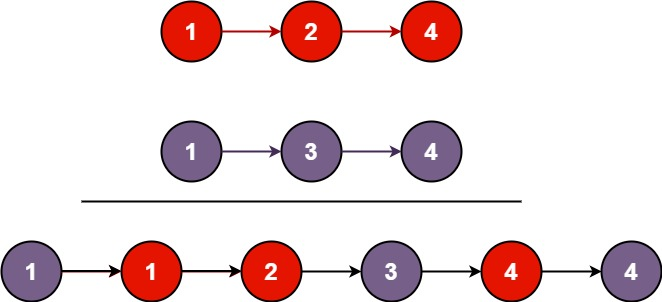Amazon

Apple

Bloomberg

Capital One

IBM

Microsoft

Oracle

VMware

Yahoo

eBay

2020-10-13

## 21. Merge Two Sorted Lists

### Question:

Merge two sorted linked lists and return it as a new sorted list. The new list should be made by splicing together the nodes of the first two lists.

#### Example 1:``````Input: l1 = [1,2,4], l2 = [1,3,4]
Output: [1,1,2,3,4,4]
``````

#### Example 2:

``````Input: l1 = [], l2 = []
Output: []
``````

#### Example 3:

``````Input: l1 = [], l2 = 
Output: 
``````

Constraints:

• The number of nodes in both lists is in the range `[0, 50]`.
• `-100 <= Node.val <= 100`
• Both `l1` and `l2` are sorted in non-decreasing order.

### Solution:

Using two pointer pointing to the head of each node, compare each value and add to the result list node.

``````/**
* Definition for singly-linked list.
* public class ListNode {
*     int val;
*     ListNode next;
*     ListNode() {}
*     ListNode(int val) { this.val = val; }
*     ListNode(int val, ListNode next) { this.val = val; this.next = next; }
* }
*/
class Solution {
public ListNode mergeTwoLists(ListNode l1, ListNode l2) {
ListNode dummy = new ListNode();
ListNode head = dummy;
while (l1 != null && l2 != null) {
if (l1.val <= l2.val) {
dummy.next = l1;
l1 = l1.next;
} else {
dummy.next = l2;
l2 = l2.next;
}
dummy = dummy.next;
dummy.next = null;
}
if (l1 != null) {
dummy.next = l1;
} else {
dummy.next = l2;
}